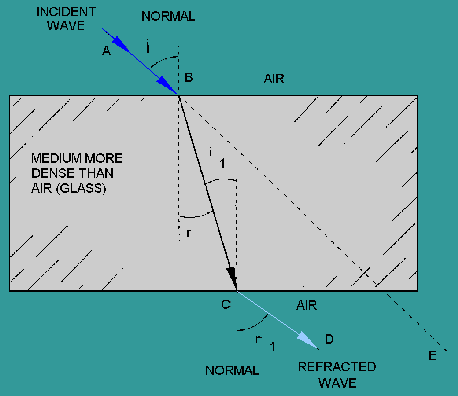Custom SearchREFRACTION OF LIGHT When a light wave passes from one medium into a medium having a different velocity of propagation (the speed waves can travel through a medium), a change in the direction of the wave will occur. This change of direction as the wave enters the second medium is called refraction. As in the discussion of reflection, the wave striking the boundary (surface) is called the incident wave, and the imaginary line perpendicular to the boundary is called the normal. The angle between the incident wave and the normal is called the angle of incidence. As the wave passes through the boundary, it is bent either toward or away from the normal. The angle between the normal and the path of the wave through the second medium is the angle of refraction. A light wave passing through a block of glass is shown in figure 2-5. The wave moves from point A to point B at a constant speed. This is the incident wave. As the wave penetrates the glass boundary at point B, the velocity of the wave is slowed down. This causes the wave to bend toward the normal. The wave then takes the path from point B to point C through the glass and becomes both the refracted wave from the top surface and the incident wave to the lower surface. As the wave passes from the glass to the air (the second boundary), it is again refracted, this time away from the normal, and takes the path from point C to point D. After passing through the last boundary, the velocity increases to the original velocity of the wave. As illustrated, refracted waves can bend toward or away from the normal. This bending depends on the velocity of the wave through different mediums. The broken line between points B and E is the path that the wave would travel if the two mediums (air and glass) had the same density. Figure 2-5. - Refraction of a wave.Another interesting condition can be shown using figure 2-5. If the wave passes from a less dense to a more dense medium, it is bent toward the normal, and the angle of refraction (r) is less than the angle of incidence (i). Likewise, if the wave passes from a more dense to a less dense medium, it is bent away from the normal, and the angle of refraction (r1) is greater than the angle of incidence (i1). An example of refraction is the apparent bending of a spoon when it is immersed in a cup of water. The bending seems to take place at the surface of the water, or exactly at the point where there is a change of density. Obviously, the spoon does not bend from the pressure of the water. The light forming the image of the spoon is bent as it passes from the water (a medium of high density) to the air (a medium of comparatively low density). Without refraction, light waves would pass in straight lines through transparent substances without any change of direction. Figure 2-5 shows that rays striking the glass at any angle other than perpendicular are refracted. However, perpendicular rays, which enter the glass normal to the surface, continue through the glass and into the air in a straight line - no refraction takes place. Q.10 A refracted wave occurs when a wave passes from one medium into another medium. What determines the angle of refraction? Q.11 A light wave enters a sheet of glass at a perfect right angle to the surface. Is the majority of the wave reflected, refracted, transmitted, or absorbed?Integrated Publishing, Inc. - A (SDVOSB) Service Disabled Veteran Owned Small Business